# Probability Worksheets

#### What is Probability?

Probability is a branch of math that calculates the likelihood of the occurrence of some given event, which is expressed as a number from 1 to 0. An event where probability is 1 can be considered a certainty. For instance, the probability of a coin toss results in either "heads" or "tails" since there is no other option. Assuming that the coin will land flat. Event with a probability of .5 can be considered to have odds of not occurring or occurring. For example, the probability of a coin toss that results in "heads" is .5 since the toss is as likely to result in "tails." The probability of 0 in an event can be considered an impossibility.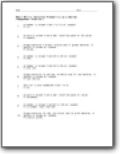###### Calculate Probability as a Decimal

You will be given a wide range of probability word problems.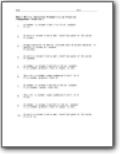###### Calculate Probability as a Fraction

You will be given a wide range of probability word problems.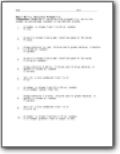###### To Two Decimal Places

Calculate the probability; write the answer as percentage rounded to two decimal places.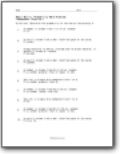###### Probability Word Problems

Determine the probability of the choice indicated by P.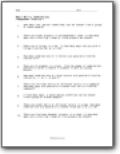###### Combinations

Students will solve word problems that center around combinations. Example: There are thirteen shirts of different colors in a cupboard. How many ways are possible to choose four shirts from the cupboard?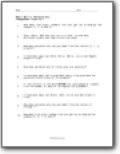###### Permutations

How many five digit numbers can you get by arranging the numbers 6, 0, 1, 5 and 2?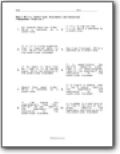###### Conditional Statements and Converses

Students breakdown these types of mathematical statements. Example: If a quadrilateral has parallel opposite sides, then it is parallelogram. Identify the hypothesis and conclusion of the conditional statement.